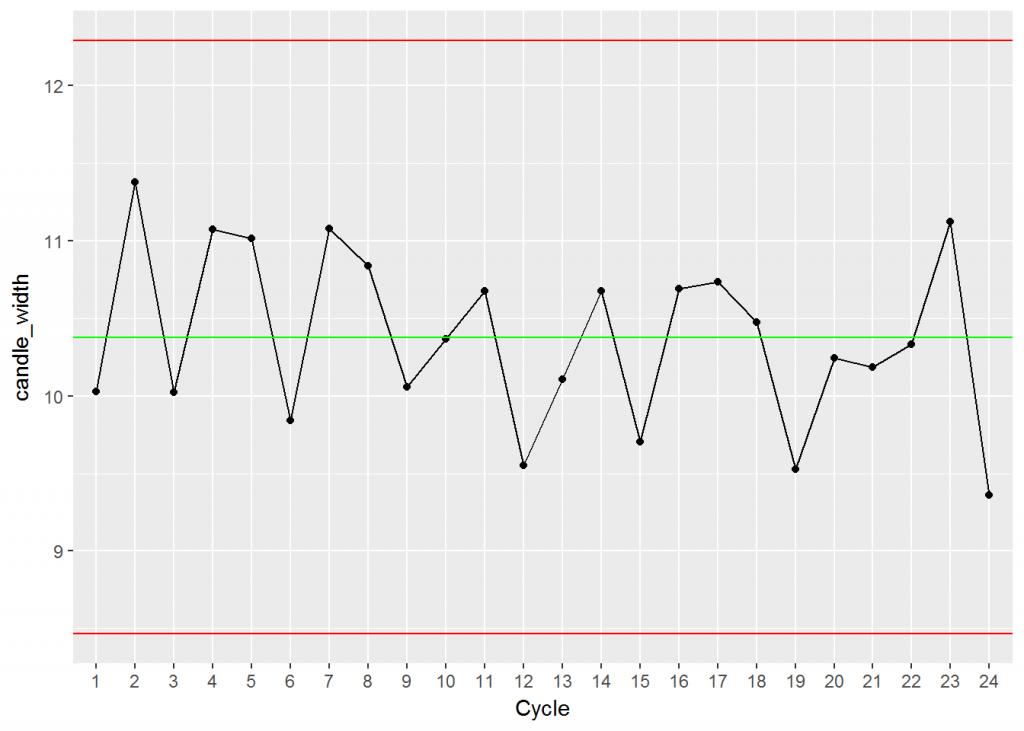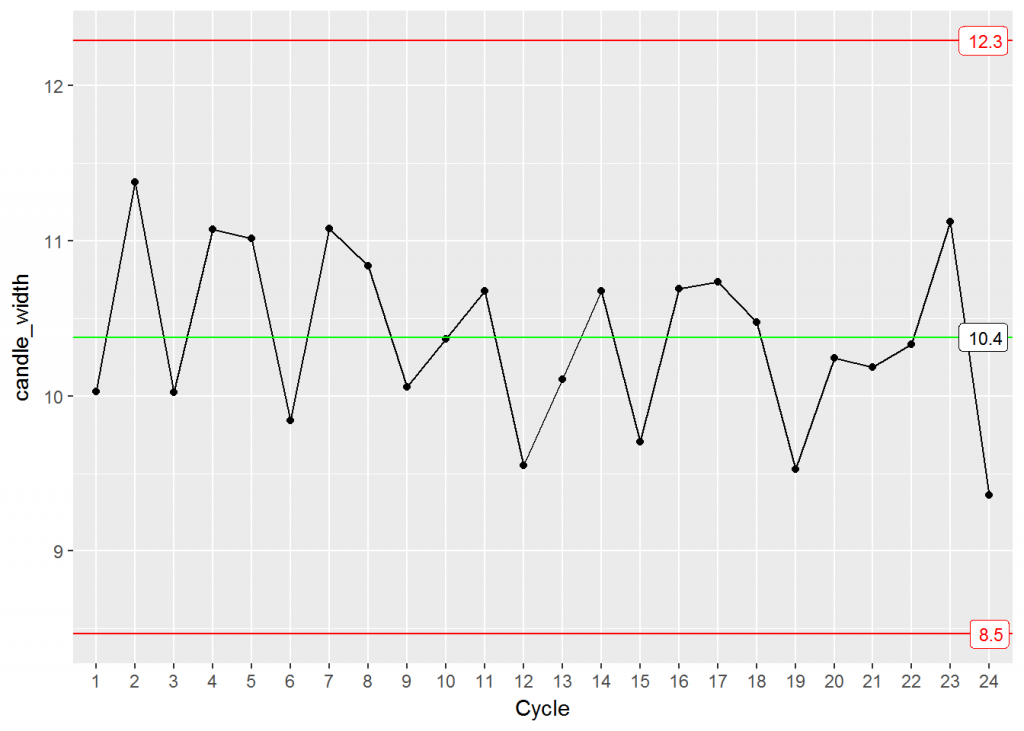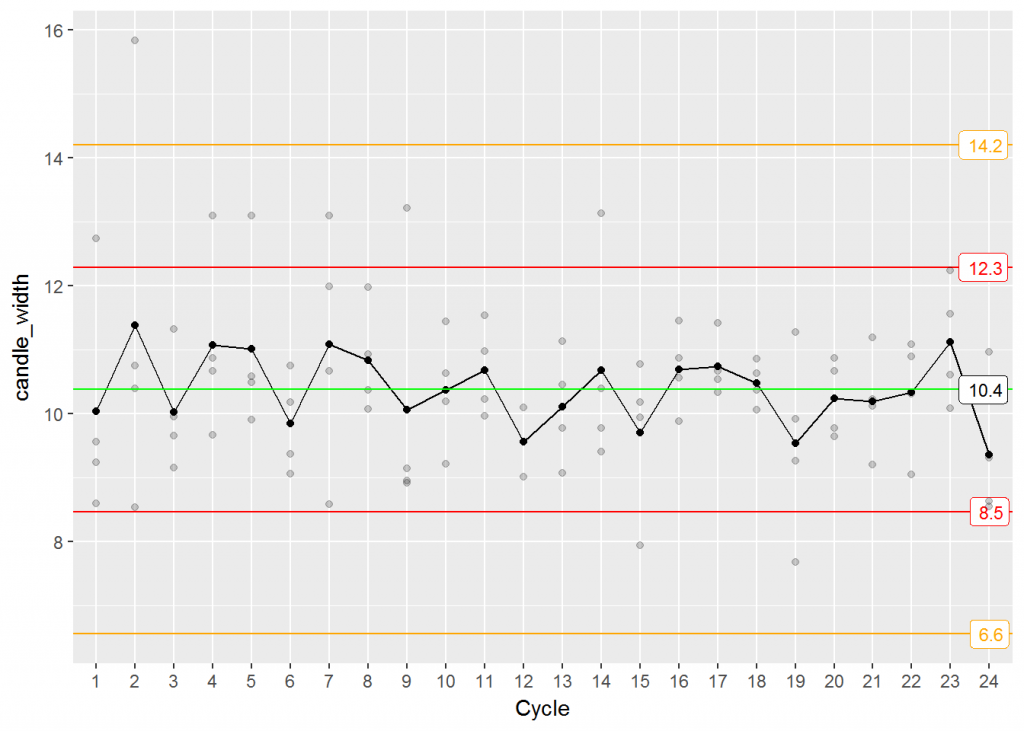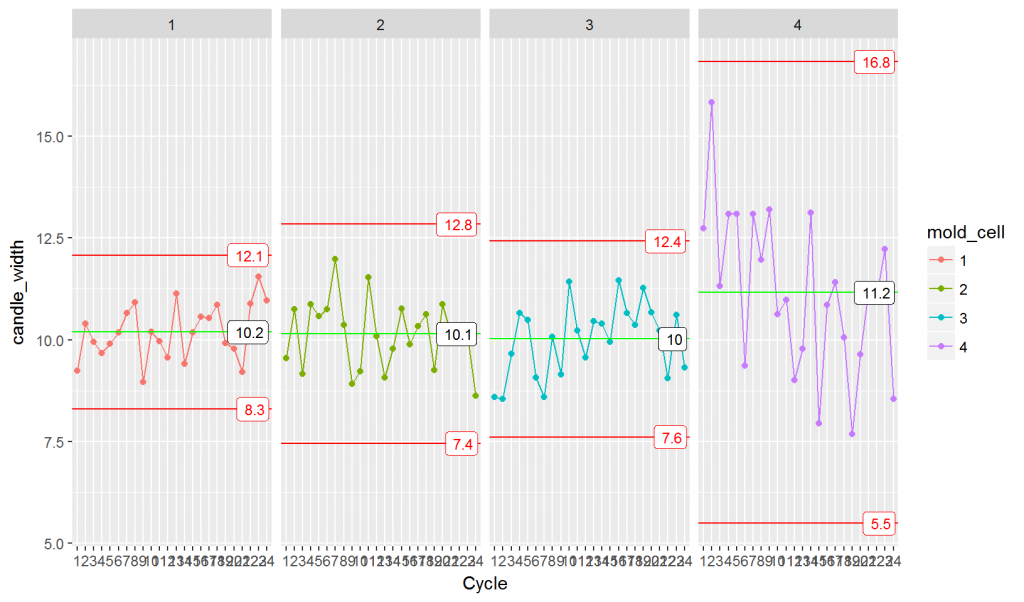XbarR charts are useful when monitoring a continuous process over time and your taking multiple samples in a given period. Some examples might include,

• the first, middle, and last parts coming off an assembly line,
• subgroups of molded parts produced several at a time over several cycles,
• batch uniformity of continuously produced chemical / material.

In this post, we will show how to make quick QC XbarR plots with the ggQC package available on cran or github.

` cran: install.package("ggQC")`

Generating an Xbar or XbarR plot with ggQC is simple. To get us started, let’s simulate some production line data on candles. The candles are shaped using a mold capable of producing 4 units a cycle. Each cycle takes an hour. Thus in a 24 hour period, the process would yield 96 candles. The parameter being tracked is candle width.

## Simulate Some Data

The code block below, generates the candle width data we are going to use to work with ggQC. Here we are generating data for the 4 mold cells. Three of the mold cells are performing normally, one of the mold cells is not.

```set.seed(5555)
candle_df1t3 <- data.frame(
Cycle = as.factor(rep(1:24, each=3)),
candle_width = rnorm(n = 3*24, mean = 10, sd = 1),
mold_cell = as.ordered(rep(1:3))
)

candle_df4 <- data.frame(
Cycle = as.factor(rep(1:24, each=1)),
candle_width = rnorm(n = 1*24, mean = 11, sd = 2),
mold_cell = as.ordered(rep(4, each=24))
)

candle_df <- rbind(candle_df1t3, candle_df4)
```

## Simple XbarR Plot

Making a plot with ggQC and ggplot is simple

NOTEs:

Remember to set the group aesthetic equal to 1. Otherwise you will end up with far more control lines than you want.

XbarR is the default method for stat_QC and stat_QC_labels functions.

```library(ggplot2)
library(ggQC)

XbarR <- ggplot(candle_df, aes(x = Cycle, y = candle_width, group = 1)) +
stat_summary(fun.y = mean, geom = "point") +
stat_summary(fun.y = mean, geom = "line") +
stat_QC()

XbarR
```Data looks to be in good control, but it would be nice to have the center line and control limits labeled before presenting it to the line manager.

## Labeled XbarR Plot

```XbarR + stat_QC_labels()
```Your line manager is happy to see the candles are being produced as intended, but would like to get a sense of the process consistency. For this you will need an R-Bar chart.

## R Bar Chart

```R_Bar <- ggplot(candle_df, aes(x = Cycle, y = candle_width, group = 1)) +
stat_summary(fun.y = QCrange, geom = "point") +
stat_summary(fun.y = QCrange, geom = "line") +
stat_QC(method="rBar") +
stat_QC_labels(method="rBar") + ylab("R-Bar")

R_Bar
```The second run for the day was more inconsistent than usual, and the line manager lifts an eye brow. He has just received a customer complaint that some of the candles are too wide to fit in their candle holders. He asks if you can show the individuals on the plot and the natural control limits.

## XbarR with Individuals

```XbarR <- ggplot(candle_df, aes(x = Cycle, y = candle_width, group = 1)) +
stat_summary(fun.y = mean, geom = "point") +
stat_summary(fun.y = mean, geom = "line") +
stat_QC() + stat_QC_labels() +
# Show Individuals
geom_point(alpha= 1/5) +
stat_QC(n=1, color.qc_limits = "orange") +
stat_QC_labels(n=1, color.qc_limits = "orange")

XbarR
```Orange lines show the 3sigma control limits for the individual samples. The line manager is surprised to see so many individuals’ widths are over 13 units, and wants to know how a candle width of nearly 16 could occur. Leaving the conversation you are asked to examine the data as a function of the different cells in the mold.

## Colorizing the Data

```XbarR <- ggplot(candle_df, aes(x = Cycle, y = candle_width, group = 1, color=mold_cell)) +
stat_summary(fun.y = mean, geom = "point") +
stat_summary(fun.y = mean, geom = "line") +
stat_QC() + stat_QC_labels() +
# Show Individuals
geom_point(alpha= 1/2) +
stat_QC(n=1, color.qc_limits = "orange") +
stat_QC_labels(n=1, color.qc_limits = "orange")

XbarR

```Mold Cell 4 (purple in the plot) looks a little suspicious. So you plot an XmR chart for each cell.

## Faceting

```XmR <- ggplot(candle_df,
aes(x = Cycle, y = candle_width, group = 1, color = mold_cell)) +
geom_point() + geom_line() +
stat_QC(method="XmR") + stat_QC_labels(method="XmR") +
facet_grid(.~mold_cell)

XmR
```Nice work! Looks like we need to replace the mold.